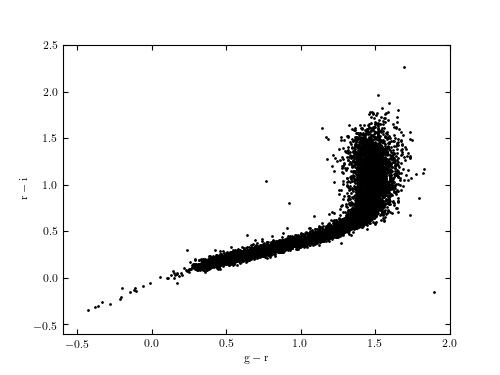# SDSS Stripe 82 Standard Stars¶

Figure 1.6.

The g-r vs. r-i color-color diagram for the first 10,000 entries in the Stripe 82 Standard Star Catalog. The region with the highest point density is dominated by main sequence stars. The thin extension toward the lower-left corner is dominated by the so-called blue horizontal branch stars and white dwarf stars.# Author: Jake VanderPlas
#   The figure produced by this code is published in the textbook
#   "Statistics, Data Mining, and Machine Learning in Astronomy" (2013)
#   To report a bug or issue, use the following forum:
from matplotlib import pyplot as plt
from astroML.datasets import fetch_sdss_S82standards

#----------------------------------------------------------------------
# This function adjusts matplotlib settings for a uniform feel in the textbook.
# Note that with usetex=True, fonts are rendered with LaTeX.  This may
# result in an error if LaTeX is not installed on your system.  In that case,
# you can set usetex to False.
if "setup_text_plots" not in globals():
from astroML.plotting import setup_text_plots
setup_text_plots(fontsize=8, usetex=True)

#------------------------------------------------------------
# Fetch the stripe 82 data
data = fetch_sdss_S82standards()

# select the first 10000 points
data = data[:10000]

# select the mean magnitudes for g, r, i
g = data['mmu_g']
r = data['mmu_r']
i = data['mmu_i']

#------------------------------------------------------------
# Plot the g-r vs r-i colors
fig, ax = plt.subplots(figsize=(5, 3.75))
ax.plot(g - r, r - i, marker='.', markersize=2,
color='black', linestyle='none')

ax.set_xlim(-0.6, 2.0)
ax.set_ylim(-0.6, 2.5)

ax.set_xlabel(r'${\rm g - r}$')
ax.set_ylabel(r'${\rm r - i}$')

plt.show()